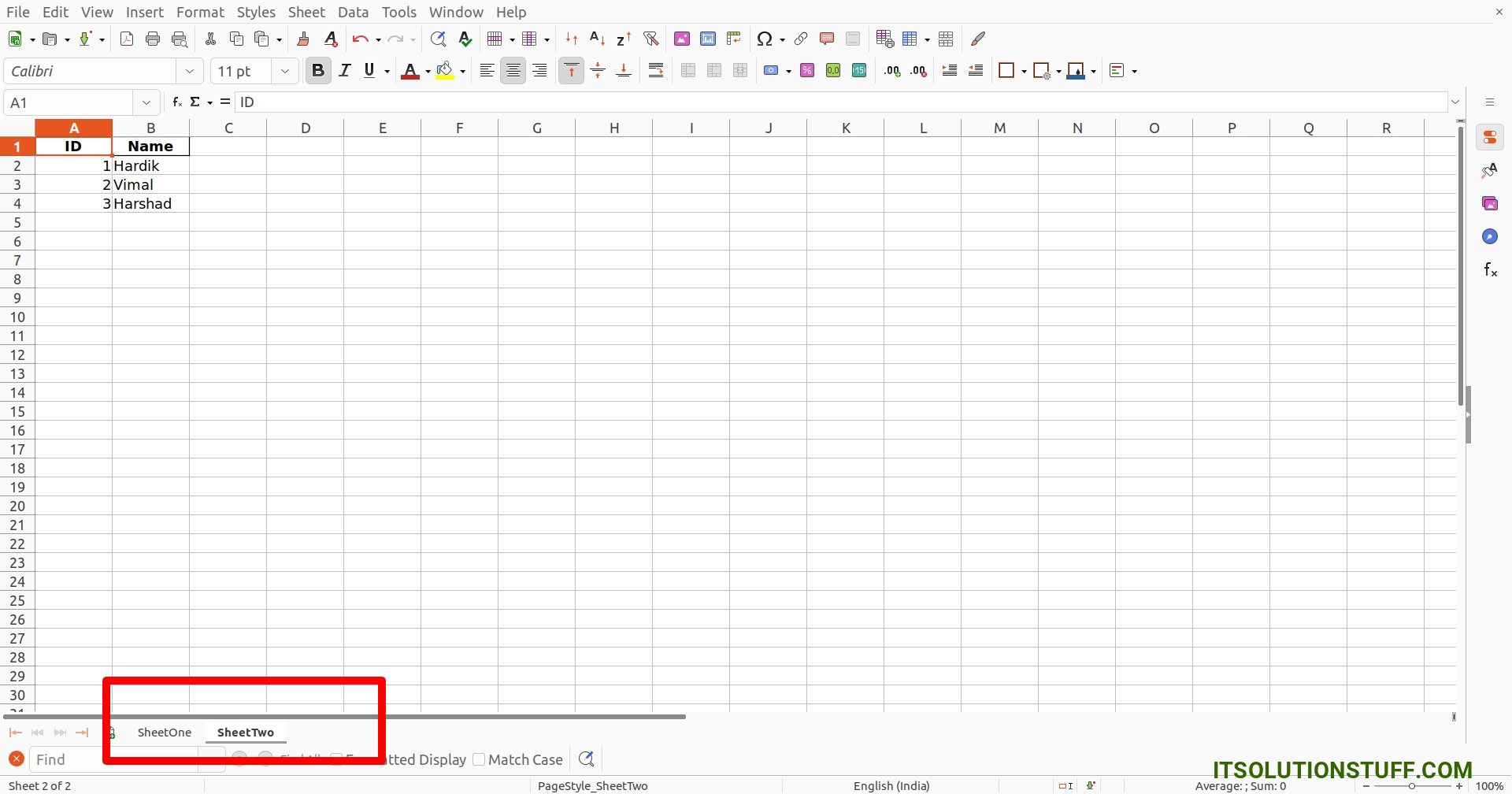# Python Pandas Create Excel File with Multiple Sheets

By Hardik Savani September 12, 2022 Category : Python

Hey,

This article is focused on python pandas create excel file with multiple sheets. I am going to show you about pandas create excel file with multiple sheets. This post will give you a simple example of pandas write to multiple excel sheets. I explained simply about python pandas create excel file with multiple sheets.

Pandas is an open source Python package. Pandas is used to analyze data. We can work with DataFrame using Python Pandas.

In this example, we will create excel file with SheetOne and SheetTwo multiple sheet. so let's see the below examples.

You can use these examples with python3 (Python 3) version.

If you haven't install pandas in your system then you can install using the below command:

```pip install pandas
```

Example 1: Multiple Sheet

main.py

```import pandas as pd

# Create a Pandas Excel writer using XlsxWriter
writer = pd.ExcelWriter('demo.xlsx', engine='xlsxwriter')

# Create SheetOne with Data
df = pd.DataFrame({'ID': [1, 2, 3],
'Name': ["Hardik Savani", "Vimal Kashiyani", "Harshad Pathak"],

df.to_excel(writer, sheet_name='SheetOne', index=False)

# Create SheetTwo with Data
df = pd.DataFrame({'ID': [1, 2, 3],

df.to_excel(writer, sheet_name='SheetTwo', index=False)

# Save Data to File
writer.save()
```

Output:

Now, It will generated demo.xlsx file in your root path with the below content.

demo.xlsx